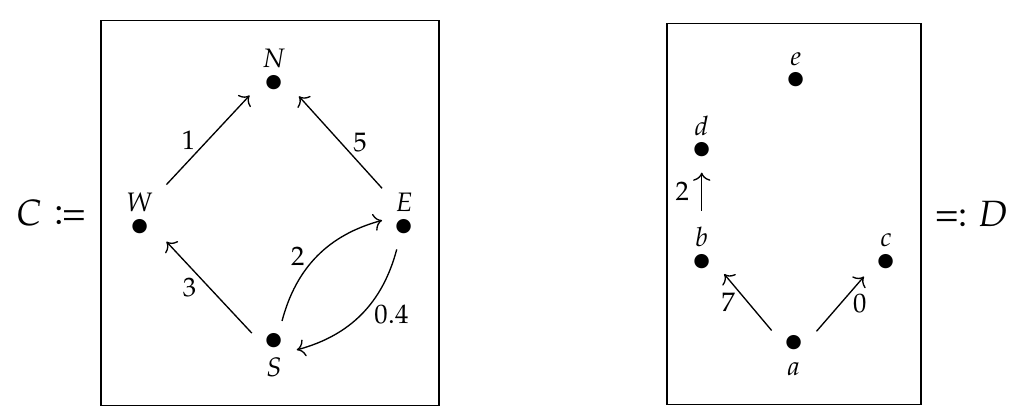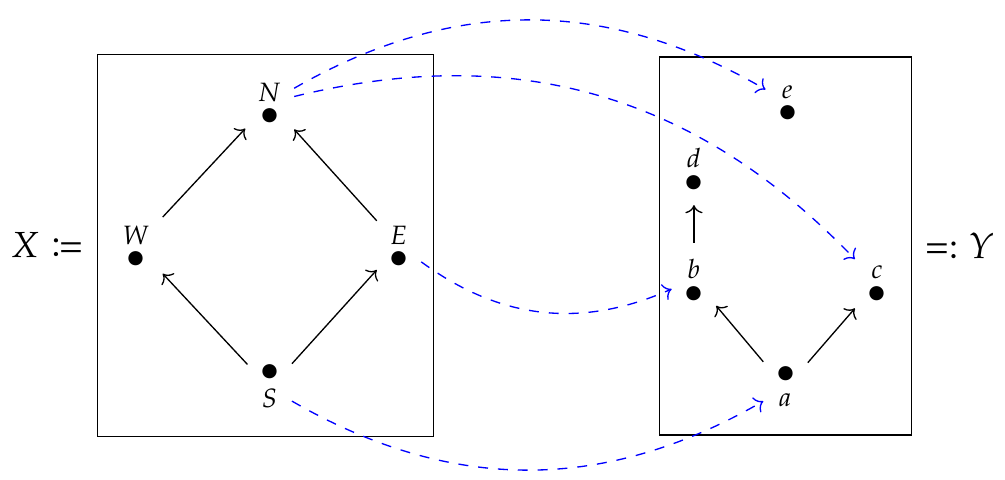Scott Oswald wrote:

> I think I am not understanding something correctly about \$$\mathbf{Cost}\$$-categories. Isn't the composition rule for \$$\mathbf{Cost}\$$-categories like this: If we have objects \$$N,W,S\$$, then the composition rule is \$$\mathcal{C}(S,W) + \mathcal{C}(W,N) \ge \mathcal{C}(S,N)\$$ so \$$4 \ge \mathcal{C}(S,N)\$$. There's another path between \$$S\$$ and \$$N\$$ via \$$E\$$, but it gives \$$7 \ge \mathcal{C}(S,N)\$$. Does this mean that \$$\mathcal{C}(S,N) = 4\$$ since it's the meet of compositions that give \$$\mathcal{C}(S,N)\$$?

I explained this stuff a bit here:

* [Lecture 33 - Chapter 2: Tying up loose ends](https://forum.azimuthproject.org/discussion/2192/lecture-33-chapter-2-tying-up-loose-ends/p1).

so if you don't remember that it might help to look at it.

You're definitely on the right track! Perhaps what's confusing you is this. These pictures:are not technically pictures of \$$\mathbf{Cost}\$$-categories; they are pictures of \$$\mathbf{Cost}\$$-weighted graphs, a concept I explained in Lecture 33. But we can _construct_ a \$$\mathbf{Cost}\$$-category from a \$$\mathbf{Cost}\$$-weighted graph, by _defining_ \$$\mathcal{C}(x,y)\$$ to be the price of the least expensive route from \$$x\$$ to \$$y\$$, i.e. the infimum over all edge paths from \$$x\$$ to \$$y\$$ of the sum of the costs labelling these edges.

So, my objection to what you said is only a technical one: we are not using the rules for composition in a \$$\mathbf{Cost}\$$-category to deduce \$$\mathcal{C}(S,N) = 4\$$; rather, we are using a specific recipe to construct a \$$\mathbf{Cost}\$$-category from a \$$\mathbf{Cost}\$$-weighted graph, and this recipe gives us \$$\mathcal{C}(S,N) = 4\$$.

> Also, it seems to me that \$$\mathcal{V}\$$-profunctors are a way of gluing together two \$$\mathcal{V}\$$-categories such that the result is a \$$\mathcal{V}\$$-category.

This is certainly one thing one can do with a \$$\mathcal{V}\$$-profunctor! Given a \$$\mathcal{V}\$$-profunctor \$$\Psi : \mathcal{X} \nrightarrow \mathcal{Y}\$$, we can glue \$$\mathcal{X}\$$ and \$$\mathcal{Y}\$$ together as you're imagining and get a new \$$\mathcal{V}\$$-category called their **collage.**

Simon Willerton explained this in the case \$$\mathcal{V} = \textbf{Bool}\$$ back in [comment #7 to Lecture 57](https://forum.azimuthproject.org/discussion/comment/19971/#Comment_19971):

>> In some sense, in the pictures it would be more sensible to put \$$Y\$$ *on top of* \$$X\$$. One can think (sometimes usefully, sometimes less usefully) of a feasability relation (or profunctor) between preorders as giving rise to a new preorder, known as the **collage**. The underlying set of the collage is the disjoint union \$$X \sqcup Y\$$ and on both \$$X\$$ and \$$Y\$$ the new preorder restricts to the preorders you started with, but for all \$$x \in X\$$ and \$$y \in Y\$$ you have \$$y\not\le x\$$ whilst \$$x\le y\, \iff\ \Phi(x,y)\$$.

> The pictures drawn with the blue arrows are the Hasse diagrams of these collages. Nothing in \$$Y\$$ ever comes before anything in \$$X\$$ in this preorder, so that's why it would make sense to draw \$$Y\$$ on top of \$$X\$$. I'll leave it for someone to produce such a version of the examples above :-).